Next: Pauli Representation Up: Spin Angular Momentum Previous: Spin Space

# Eigenstates of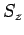and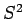Since the operatorsandcommute, they must possess simultaneous eigenstates (see Sect. 4.10). Let these eigenstates take the form [see Eqs. (556) and (557)]: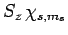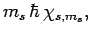(717)(718)

Now, it is easily demonstrated, from the commutation relations (711) and (712), that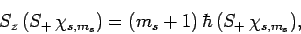(719)

and(720)

Thus,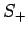and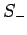are indeed the raising and lowering operators, respectively, for spin angular momentum (see Sect. 8.4). The eigenstates ofandare assumed to be orthonormal: i.e.,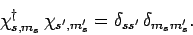(721)

Consider the wavefunction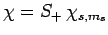. Since we know, from Eq. (713), that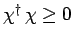, it follows that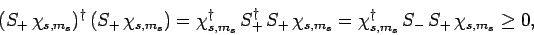(722)

where use has been made of Eq. (708). Equations (710), (717), (718), and (721) yield(723)

Likewise, ifthen we obtain(724)

Assuming that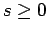, the above two inequalities imply that(725)

Hence, at fixed, there is both a maximum and a minimum possible value thatcan take.

Letbe the minimum possible value of. It follows that (see Sect. 8.6)(726)

Now, from Eq. (709),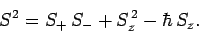(727)

Hence,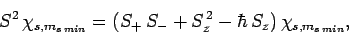(728)

giving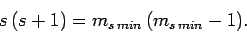(729)

Assuming that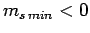, this equation yields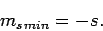(730)

Likewise, it is easily demonstrated that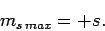(731)

Moreover,(732)

Now, the raising operator, acting upon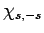, converts it into some multiple of. Employing the raising operator a second time, we obtain a multiple of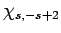. However, this process cannot continue indefinitely, since there is a maximum possible value of. Indeed, after acting upona sufficient number of times with the raising operator, we must obtain a multiple of, so that employing the raising operator one more time leads to the null state [see Eq. (732)]. If this is not the case then we will inevitably obtain eigenstates ofcorresponding to, which we have already demonstrated is impossible.

It follows, from the above argument, that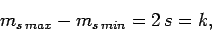(733)

whereis a positive integer. Hence, the quantum numbercan either take positive integer or positive half-integer values. Up to now, our analysis has been very similar to that which we used earlier to investigate orbital angular momentum (see Sect. 8). Recall, that for orbital angular momentum the quantum number, which is analogous to, is restricted to take integer values (see Cha. 8.5). This implies that the quantum number, which is analogous to, is also restricted to take integer values. However, the origin of these restrictions is the representation of the orbital angular momentum operators as differential operators in real space (see Sect. 8.3). There is no equivalent representation of the corresponding spin angular momentum operators. Hence, we conclude that there is no reason why the quantum numbercannot take half-integer, as well as integer, values.

In 1940, Wolfgang Pauli proved the so-called spin-statistics theorem using relativistic quantum mechanics. According to this theorem, all fermions possess half-integer spin (i.e., a half-integer value of), whereas all bosons possess integer spin (i.e., an integer value of). In fact, all presently known fermions, including electrons and protons, possess spin one-half. In other words, electrons and protons are characterized byand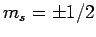.Next: Pauli Representation Up: Spin Angular Momentum Previous: Spin Space
Richard Fitzpatrick 2010-07-20